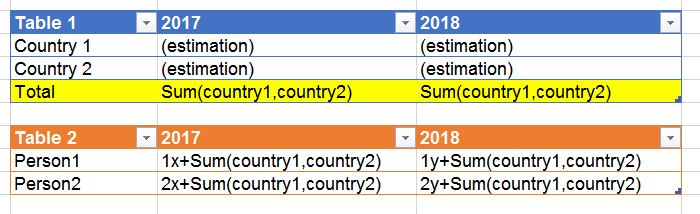# Qlik Sense App Development

HighlightedPartner

## Using estimated value from another table

Hello everyone

I need very much help with this estimation.

I have Table 1 and Table 2.

• Year- dimension
• Two measures: Country 1 and Country 2Table 2 uses Total values from Table 1 estimating values.

I cannot find how I can get Total value (From Table 1) calculating Table 2 without typing full formula that is used in Table 1. Is it possible somehow to refer to the Total value? Maybe there is any formula to get the Total value?

I cannot type in Table 2 full formula from Table 1, because I do not have enough space in the script as both Tables are much more complicated than the example.

5 Replies
Valued Contributor

## Re: Using estimated value from another table

Hi Ignas,

I assume you want to do it in script, so would it be possible to make a LEFT JOIN from a temporary table resulting as an aggregation of Table1 (eg group by year) to TABLE 2?

Regards,

HPartner

## Re: Using estimated value from another table

Hello Muñoz,

Thank you for your response. I am not sure if it is possible, because tables are created in QS visualization (Pivot table) and the estimation is also done in the table.

Would it be possible if I share with you the App and you could have a look? I could send to your email, if you have few minutes to lookRegards,

Ignas

Valued Contributor

## Re: Using estimated value from another table

Sure!

Regards,

HPartner

## Re: Using estimated value from another table

Would you tell me your email please? I cannot find it in your profile. Thanks a lot

Valued Contributor

## Re: Using estimated value from another table

Hi Ignas,

I assume that Year dimension is shared (is the same) by Table1 and Table2 and each person works in a country, so the Table2 expression formula would be something like:

Sum([Personal Portion]) + Sum(TOTAL <Country> Estimation)

Hope it serves! I think that the key of your problem is the TOTAL modifier. Take a look at it into the Reference Manual...

Regards,
H